Download Presentation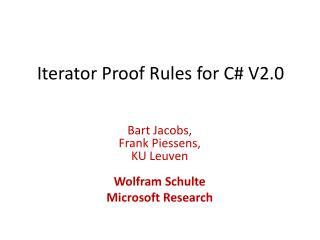Iterator Proof Rules for C# V2.0

# Iterator Proof Rules for C# V2.0 - PowerPoint PPT Presentation

Iterator Proof Rules for C# V2.0. Bart Jacobs, Frank Piessens, KU Leuven Wolfram Schulte Microsoft Research. Outline. Iterators in C# How to specify and verify iterators and foreach loops? How to prevent interference between iterators and foreach loops?. The Iterator pattern in C# 2.0.I am the owner, or an agent authorized to act on behalf of the owner, of the copyrighted work described.
Download Presentation## Iterator Proof Rules for C# V2.0

An Image/Link below is provided (as is) to download presentation

Download Policy: Content on the Website is provided to you AS IS for your information and personal use and may not be sold / licensed / shared on other websites without getting consent from its author.While downloading, if for some reason you are not able to download a presentation, the publisher may have deleted the file from their server.

- - - - - - - - - - - - - - - - - - - - - - - - - - E N D - - - - - - - - - - - - - - - - - - - - - - - - - -
Presentation Transcript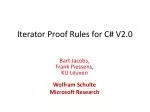### Iterator Proof Rules for C# V2.0

Bart Jacobs,Frank Piessens,KU Leuven Wolfram Schulte

Microsoft Research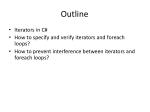Outline
• Iterators in C#
• How to specify and verify iterators and foreach loops?
• How to prevent interference between iterators and foreach loops?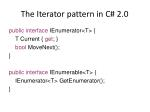The Iterator pattern in C# 2.0

public interface IEnumerator<T> {

T Current { get; }

bool MoveNext();

}

public interface IEnumerable<T> {

IEnumerator<T> GetEnumerator();

}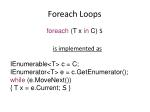Foreach Loops

foreach (T x in C) S

is implemented as

IEnumerable<T> c = C;

IEnumerator<T> e = c.GetEnumerator();

while (e.MoveNext())

{ T x = e.Current; S }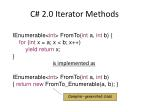C# 2.0 Iterator Methods

IEnumerable<int> FromTo(int a, int b) {

for (int x = a; x < b; x++)

yield return x;

}

is implemented as

IEnumerable<int> FromTo(int a, int b)

{ return new FromTo_Enumerable(a, b); }

Compiler-generated class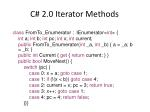C# 2.0 Iterator Methods

class FromTo_Enumerator : IEnumerator<int> {

int a; int b; int pc; int x; int current;

public FromTo_Enumerator(int _a, int _b) { a = _a; b = _b; }

public int Current { get { return current; } }

public bool MoveNext() {

switch (pc) {

case 0: x = a; goto case 1;

case 1: if (!(x < b)) goto case 4;

case 2: current = x; pc = 3; return true;

case 3: x++; goto case 1;

case 4: pc = 4; return false;

}}}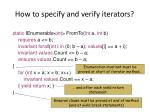How to specify and verify iterators?

static IEnumerable<int> FromTo(int a, int b)

requires a <= b;

invariantforall{int i in (0; b – a); values[i] == a + i};

invariantvalues.Count <= b – a;

ensuresvalues.Count == b – a;

{

for (int x = a; x < b; x++)

invariantvalues.Count == x – a;

{ yield return x; }

}

Enumeration invariant must be proved at start of iterator method…

… and after each yield return statement.

Ensures clause must be proved at end of method (and at yield break statements)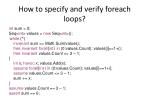How to specify and verify foreach loops?

int sum = 0;

Seq<int> values = new Seq<int>();

foreach (int x in FromTo(1, 3))

invariant sum == Math.Sum(values);

free invariantforall{int i in (0:values.Count); values[i]==1+i};

free invariant values.Count <= 3 – 1;

{

int x; havoc x; values.Add(x);

assumeforall{int i in (0:values.Count); values[i]==1+i};

assume values.Count <= 3 – 1;

sum += x;

}

assume values.Count == 3 – 1;

assert sum == 6;

while (*)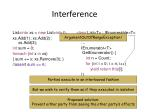Interference

List<int> xs = new List<int>();

int sum = 0;

foreach (int x in xs)

{ sum += x; xs.Remove(0); }

//assert sum == 6;

class List<T> : IEnumerable<T> {

IEnumerator<T> GetEnumerator() {

int n = Count;

for (int i = 0; i < n; i++)

yield returnthis[i];

}}

ArgumentOutOfRangeException !

Parties execute in an interleaved fashion

But we wish to verify them as if they executed in isolation

Proposed solution:

Prevent either party from seeing the other party’s effects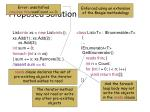Enforced using an extension of the Boogie methodology

Error: unsatisfiedrequiresthis.readCount == 0;

Proposed Solution

List<int> xs = new List<int>();

int sum = 0;

foreach (int x in xs)

{ sum += x; xs.Remove(0); }

//assert sum == 6;

class List<T> : IEnumerable<T> {

IEnumerator<T> GetEnumerator()

int n = Count;

for (int i = 0; i < n; i++)

yield returnthis[i];

}}

reads clause declares the set ofpre-existing objects the iterator method wishes to read

And the foreach loop body may not write the objects in the reads clause

The iterator method may not read or write any other pre-existing objectsAdding read-only objects to the Boogie methodology
• Each object gets three special fields:
• bool inv;
• bool writable;
• int readCount; // never negative
• o.f = x; requires

o.writable && o.readCount == 0 && !o.inv

• x = o.f; requires

o.writable || 0 < o.readCountmeans

assert o.writable || 0 < o.readCount;

assert o.inv;

foreach ([Owned] field f of o)

S

foreach ([Owned] field f of o)

partial class List<T> :

IEnumerable<T> {

IEnumerator<T> GetEnumerator()

int n = Count;

for (int i = 0; i < n; i++)

yield returnthis[i];

}}

partial class List<T> {

[Owned] T[] elems;

T this[int index] {

get

requires

inv &&

(writable ||